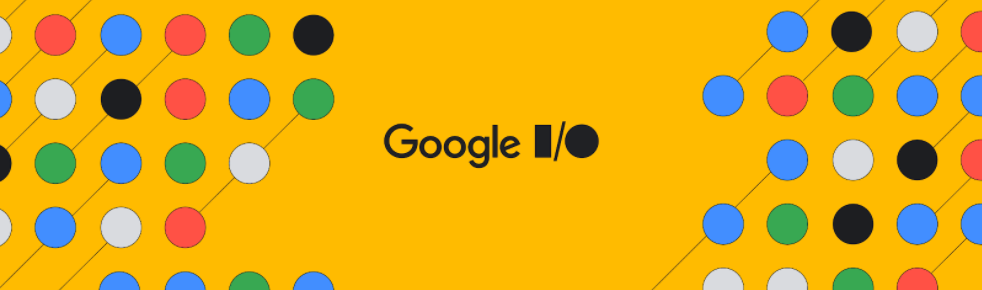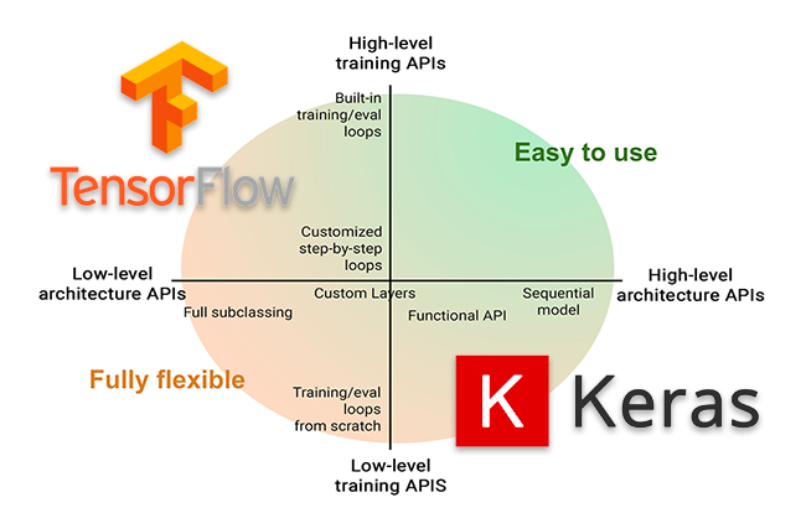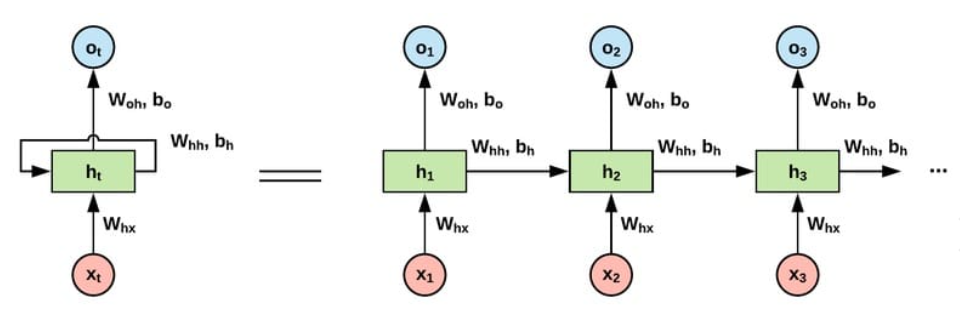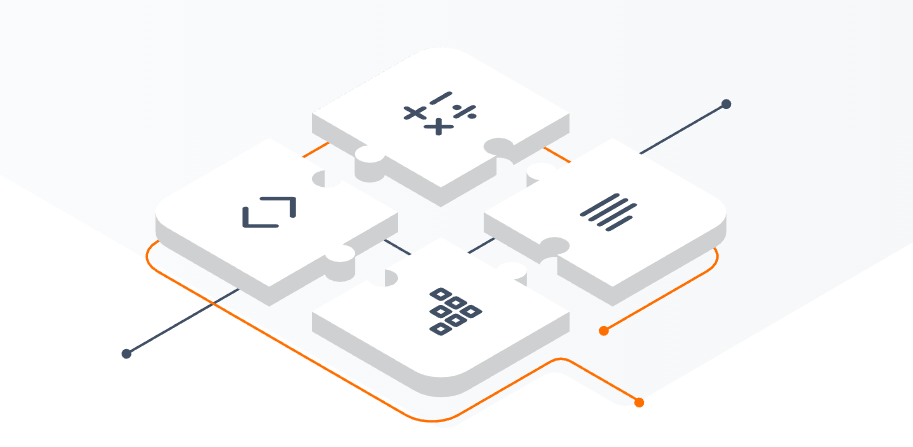# TensorFlow与Keras：强大而简洁的机器学习组合### 一 . TensorFlow简介

• 代码示例：构建一个简单的线性回归模型

``````import tensorflow as tf
​
# 定义训练数据
x_train = [1, 2, 3, 4, 5]
y_train = [2, 4, 6, 8, 10]
​
# 定义模型结构
model = tf.keras.Sequential()
​
# 编译模型
model.compile(optimizer='sgd', loss='mean_squared_error')
​
# 训练模型
model.fit(x_train, y_train, epochs=100)
​
# 预测
x_test = [6, 7, 8, 9, 10]
y_pred = model.predict(x_test)
print(y_pred)``````

### 二 . TensorFlow高级API —— KerasKeras是一个TensorFlow高级的神经网络API，为开发者提供了一种简单而直观的方式来构建深度学习模型。它建立在TensorFlow等后端引擎之上，提供了丰富的模型构建和训练功能，使得快速原型设计和实验变得更加容易。

• 代码示例：使用Keras构建一个简单的卷积神经网络模型

``````import tensorflow as tf
from tensorflow.keras import layers
​
# 构建模型
model = tf.keras.Sequential()
model.add(layers.Conv2D(32, (3, 3), activation='relu', input_shape=(28, 28, 1)))
​
# 编译模型
​
# 加载数据
(x_train, y_train), (x_test, y_test) = tf.keras.datasets.mnist.load_data()
​
# 数据预处理
x_train = x_train.reshape((60000, 28, 28, 1))
x_train = x_train.astype('float32') / 255
x_test = x_test.reshape((10000, 28, 28, 1))
x_test = x_test.astype('float32') / 255
​
# 训练模型
model.fit(x_train, y_train, epochs=5, batch_size=64)
​
# 评估模型
test_loss, test_acc = model.evaluate(x_test, y_test)
print('Test accuracy:', test_acc)``````

• 代码示例 ： 文本分类``````import tensorflow as tf
from tensorflow.keras.datasets import imdb
from tensorflow.keras.models import Sequential
from tensorflow.keras.layers import Dense, Embedding, LSTM
from tensorflow.keras.preprocessing import sequence
​
# 加载IMDB电影评论数据集
(x_train, y_train), (x_test, y_test) = imdb.load_data(num_words=5000)
​
# 限制评论长度并进行填充
max_words = 500
​
# 构建LSTM模型
model = Sequential()
​
# 编译模型
model.compile(loss='``````

### 三. 使用 KerasCV 和 KerasNLP 应用机器学习KerasCV是一个用于计算机视觉任务的库，它基于Keras并提供了许多预训练的计算机视觉模型和工具函数。下面是一个使用KerasCV进行图像分类的示例。

• 代码示例：使用KerasCV进行图像分类

``````from keras_cv import load_model, get_stacked_model
import numpy as np
​
# 加载CIFAR-10数据集
(x_train, y_train), (x_test, y_test) = load_batch("cifar10")
​
# 数据预处理
x_train = x_train.astype('float32') / 255
x_test = x_test.astype('float32') / 255
​
# 加载预训练的ResNet50模型
​
# 进行图像分类预测
preds = model.predict(x_test)
predicted_labels = np.argmax(preds, axis=1)
​
# 输出预测结果
print(predicted_labels)
​``````

...全文
37 回复 打赏 收藏 转发到动态 举报Python机器学习与数据挖掘day18huggingface实操_Transformers是TensorFlow 2.0和PyTorch的最新自然语言处理库【上】
transformers作者|huggingface编译|VK来源|GithubTransformers(以前称为pytorch-transformers和pytorch-pretrained-bert)提供用于自然语言理解(NLU)和自然语言生成(NLG)的最先进的模型(特性与pytorch-transformers一样易于使用像Keras一样强大简洁在NLU和NLG任务上具有高性能教育者和从...WandB 工具的 “MissingSectionHeaderError” Bugfix
WandB，也简称W&B，是一个用于机器学习项目的开源工具，可以帮助用户跟踪、可视化和优化模型训练过程。wandb 可以与各种流行的机器学习框架和库集成，如 TensorFlow, PyTorch, Keras, Scikit-learn 等，也可以支持自定义的训练脚本。WandB 是一个强大而灵活的工具，可以帮助用户提高机器学习项目的效率和质量。之后，仍然报错 MissingSectionHeaderError。下是否已存有账户信息，如有，可删除。kerod:DETR-在Tensorflow 2中更快地实现RCNN
KEROD-TensorFlow 2.X的对象检测（FasterRCNN，DeTr） - Kerod是针对生产的目标检测算法（Faster R-CNN，DeTr）的纯tensorflow 2实现。 它代表Keras对象检测。 它旨在为Tensorflow 2.X构建清晰，可重用，经过测试，简单且文档化的代码库。 许多想法都基于， 和 。 特征 像Keras一样强大简洁 对教育者和从业者的进入门槛低 在训练和推理中处理批处理 丰富的 多GPU Mixed_precision。 您可以在此尝试 无需费劲下载COCO或PascalVoc。 我们只使用了tensorflow_datasets 简单（再次） 演算法 DeTr from： 单尺度SMCA（WIP并非本文的确切实现方式的100％）： Mask-RCNN （很多零件已经在这里，只需要将所有内容放在一起即可。很快就会到来。tensorflow实践（一） 安装和调试

1,278发帖与我相关我的任务
android 企业社区• 近7日
• 近30日
• 至今